# Coloring the Odd Distance Graph

 Importance: High ✭✭✭
 Author(s): Rosenfeld, Moshe
 Subject: Graph Theory » Coloring » » Vertex coloring
 Keywords: coloring geometric graph odd distance
 Posted by: mdevos on: October 3rd, 2007

The Odd Distance Graph, denoted, is the graph with vertex set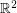and two points adjacent if the distance between them is an odd integer.

Question   Is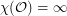?

This question is a relative of the famous problem about coloring the Unit Distance Graph (the graph onwhere two points are adjacent if the distance between them is 1). See Moshe's online lecture Famous and lesser known problems in “elementary” combinatorial geometry and number theory at time 15:20 for a nice introduction.

Perhaps the first property ofto determine is the size of the largest complete subgraph (wereto contain arbitrarily large complete subgraphs, its chromatic number would be). It is obvious thatcontains triangles, but perhaps surprisingly, it does not contain a complete subgraph on four vertices. In other words, there do not exist four points inso that all pairwise distances are odd. This was a problem on the Putnam Exam in 1993, and is proved by Rosenfeld in [R1] and [R2].

A natural strengthening of the above question is to ask if there exists a proper-coloringso thatis a measurable set for every. Such colorings are called measurable colorings, and interestingly, the Odd Distance Graph has no finite measurable coloring. This follows from immediately from a theorem of Furstenberg, Katznelson and Weiss [FKW] which asserts that for every measurable subsetwith positive upper density, there exists a numberso that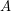contains a pair of points at distance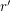for every. This theorem has a number of independent proofs, see also Falconer and Marstrand [FM], Bourgain [Bo], and Bukh [Bu].

All that seems to be known about the (usual) chromatic number ofis that.

## Bibliography

[Bo] J. Bourgain, A Szemerédi type theorem for sets of positive density in. Israel J. Math. 54 (1986), no. 3, 307--316. MathSciNet

[Bu] B. Bukh, Measurable sets with excluded distances.

[FM] K. J. Falconer and J. M. Marstrand, Plane sets with positive density at infinity contain all large distances. Bull. London Math. Soc. 18 (1986), no. 5, 471--474. MathSciNet

[FKM] H. Furstenberg, Y. Katznelson, and B. Weiss, Ergodic theory and configurations in sets of positive density. Mathematics of Ramsey theory, 184--198, Algorithms Combin., 5, Springer, Berlin, 1990. MathSciNet

[R1] M. Rosenfeld, Odd integral distances among points in the plane. Geombinatorics 5 (1996), no. 4, 156--159. MathSciNet

[R2] M. Rosenfeld Famous and lesser known problems in “elementary” combinatorial geometry and number theory (video lecture - time 15:20)

* indicates original appearance(s) of problem.

### The Odd-Distance Graph

In the book Research Problems in Discrete Geometry, on page 252, it is stated that every K_4 free graph is a subgraph of the odd distance graph. We just proved that W_5, the five wheel is not a subgraph of the odd distance graph. I further believe that there are triangle free graphs that are not subgraphs of the odd distance graph, and even graphs with large girth.

### Flaw

Actually, it appears there is a flaw with the below proof. The spectral bound on the chromatic number assumes a measurable coloring, as we want to say the following: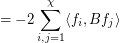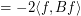but this assumes that each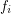is Lesbegue integrable, which in turn requires measurable coloring classes.

### Solution

I believe I have a solution. I will sketch it here. (Sorry, it's broken up into three posts because I cannot figure out how to post something more than 1000 characters...but I have seen longer solutions posted elsewhere so I assume it's okay; if not, I apologize.)

Consider the operator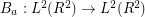defined byThis is in some sense a weighting of the adjacency operator. We can then prove the result (well-known for finite graphs) that, whereare the sup and inf of the spectrum of.

We note that the eigenfunctions ofare simply the exponential maps.

### Solution (continued)

We see that the eigenvalue of the eigenfunction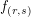is given byfor an appropriately chosen. Thus we need only actually consider, which we from now on denote. Then some calculation gives us that the integral isWe can show that (and this will suffice) that whenis near,### Solution (final part)

Let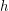be the function we are integrating. Letdenote the region for which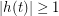and that contains the value ofwhere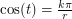. Then we note thatand the sines of the integral alternate, so we can just calculate the first one and everything else will be bounded (in particular by). With a bit of Taylor approximation, we can bound the size of eachby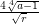, and noting thatis always positive for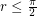, we can replace thewithand then boundby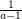. This gives us the bound we claimed above and we are done.

Jacob Steinhardt

### Circular chromatic number of the odd distance graph

The proof forhas been recently extended to, which implies the previous result, where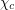is the circular chromatic number.

Nicolas Roussel.

### Correction of previous comment

The proof is actually for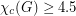.

NR.

### Subgraph construction

For any rational, there is a subgraphof the odd-distance graph with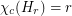Pan Zhi-Shi, Roussel Nicolas, Subgraphs of the odd-distance graph with given circular chromatic number, manuscript

NR.